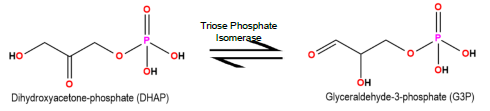Clutch Prep is now a part of Pearson
Ch. 6 - Enzymes and Enzyme KineticsWorksheetSee all chapters

# Calculating Vmax

See all sections
Sections
Enzymes
Enzyme-Substrate Complex
Lock and Key Vs. Induced Fit Models
Optimal Enzyme Conditions
Activation Energy
Types of Enzymes
Cofactor
Catalysis
Electrostatic and Metal Ion Catalysis
Covalent Catalysis
Reaction Rate
Enzyme Kinetics
Rate Constants and Rate Law
Reaction Orders
Rate Constant Units
Initial Velocity
Vmax Enzyme
Km Enzyme
Michaelis-Menten Assumptions
Michaelis-Menten Equation
Lineweaver-Burk Plot
Michaelis-Menten vs. Lineweaver-Burk Plots
Shifting Lineweaver-Burk Plots
Calculating Vmax
Calculating Km
Kcat
Specificity Constant

Concept #1: Calculating Vmax

Concept #2: Recall: Initial Velocity and Vmax

Example #1: Calculate the maximum reaction velocity (Vmax) of an enzyme if the Km = 7 mM and the initial reaction velocity(V0) = 86.71 μM/sec when the [S] = 25 mM.

Practice: Suppose an enzyme (MW = 5,000 g/mole) has a concentration of 0.05 mg/L. If the kcat is 1 x 104 s-1, what is the theoretical maximum reaction velocity for the enzyme?

Practice: For a Michaelis-Menten enzyme, what is the value of Vmax if at 1/10 Km, the V0 = 1 μmol/min.

Practice: Carbonic anhydrase catalyzes the hydration of CO2. The Km of carbonic anhydrase for CO2 is 12 mM. The initial velocity (V0) of the enzyme-catalyzed reaction was 4.5 μmole*mL-1*sec-1 when [CO2] = 36 mM. Calculate the Vmax of carbonic anhydrase.

Practice: Triose phosphate isomerase catalyzes the conversion of dihydroxyacetone phosphate (DHAP) to glyceraldehyde-3-phosphate (G3P) during glycolysis; however, this is a reversible reaction. The Km of the enzyme for G3P is 1.8 x 10 -5 M. When [G3P] = 30 μM, the initial rate of the reaction (V0) = 82.5 μmole*mL-1*sec-1. Calculate the Vmax.